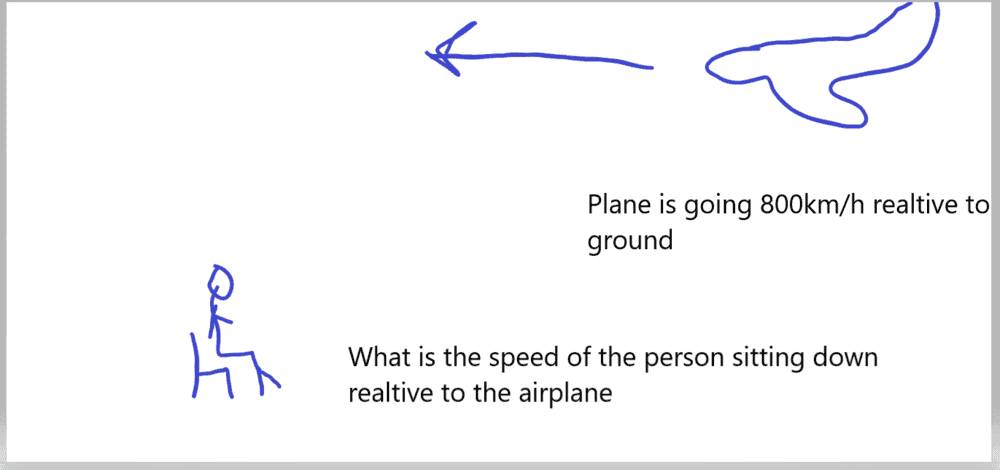# Relative Motion Comparing my motion vs an airplane

Robloxian642
Homework Statement:
What would be my relative velocity be if I compared to myself sitting vs a airplane going 800km/h
Relevant Equations:
im sitting plane moving
I'm having trouble understanding the plane one

This is what I understand so far v

I'm sitting at a chair right now and if I compare my relative motion to the ground my relative velocity would be 0m/s but if I compare my motion to the solar system I would be moving very fast. I just can't grasp my relative motion if I compare myself to a airplane

So if I compared myself to the airplane would my relative velocity be -800km/h

Gold Member
Homework Statement:: What would be my relative velocity be if I compared to myself sitting vs a airplane going 800km/h
Well, you haven't specified
1. where you're sitting, or
2. what reference point the plane is moving 800km/h relative to.

...if I compare my motion to the solar system I would be moving very fast..."
The solar system is not a discrete object. You can't really compare its motion without specifying a reference point.

So if I compared myself to the airplane would my relative velocity be -800km/h
Where does the minus symbol come from? You'd need to specify what direction the plane is going.

All that aside, what exactly are you having trouble with?

If you are sitting in a chair on the ground (i.e. on Earth), can you even say how fast the aircraft is moving without specifying what that speed is relative to? (Hint: no, you can't.)

•Robloxian642
Homework Helper
Gold Member
Each one of those velocities that you mention can be considered vectors; therefore, any relative velocity should be the result of a vectorial addition, which will result in one vector velocity that has direction and magnitude.

•Robloxian642
Robloxian642
im still very new to this material I drew out a diagram on what I wanted to figure out. This is not a homework question just something out of curiosity

I just want a easier way to think of relative motionHomework Helper
im still very new to this material I drew out a diagram on what I wanted to figure out. This is not a homework question just something out of curiosity

I just want a easier way to think of relative motion

View attachment 273014
Clearly the plane is going at -800 km/h relative to the ground. See which way the man in the chair is facing? If he turned around, then the plane would be going +800 km/h.

Possibly it would help to get back to basics. Draw a coordinate system on the ground. Label it in kilometers. Figure out where the plane is at time zero. Figure out where the plane is at time time 10 seconds. Divide the difference in position by the difference in time. That is how velocity is defined.

Now repeat it for the plane. Draw a coordinate system anchored to the plane. Figure out where the man is at time zero according to that coordinate system. Figure out where the man is at time 10 seconds. Divide.

Gold Member
Clearly the plane is going at -800 km/h relative to the ground. See which way the man in the chair is facing? If he turned around, then the plane would be going +800 km/h.
Note that this is an implicit convention.

We all accept that positive velocity is defined in this diagram by the direction of the guy in the chair is facing. But there's no objective reason for it to be so.

Last edited:
•jbriggs444
Homework Helper
Gold Member
2022 Award
Not that this is an implicit convention.
"Note that ..."?

•DaveC426913